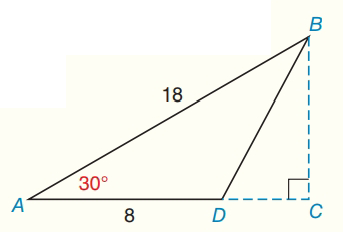Chapter 8.CR, Problem 15CR### Elementary Geometry for College St...

6th Edition
Daniel C. Alexander + 1 other
ISBN: 9781285195698

#### Solutions

Chapter
Section### Elementary Geometry for College St...

6th Edition
Daniel C. Alexander + 1 other
ISBN: 9781285195698
Textbook Problem
1 views

# Find the area of Δ A B D as shown.To determine

To find:

The area of ABD

Explanation

Theorem:

The area A of a triangle whose base has length b and whose corresponding altitude has length h is given by

A=12bh

Calculation:

Sketch the triangle as follows:

The given triangle is 30-60-90-degree right triangle.

To find BC:

BC=12AB=12×18

### Still sussing out bartleby?

Check out a sample textbook solution.

See a sample solution

#### The Solution to Your Study Problems

Bartleby provides explanations to thousands of textbook problems written by our experts, many with advanced degrees!

Get Started

#### In Problems 11-14, find the particular solution.

Mathematical Applications for the Management, Life, and Social Sciences

#### In Exercises 21-24, find the distance between the given points. 22. (1, 0) and (4, 4)

Applied Calculus for the Managerial, Life, and Social Sciences: A Brief Approach

#### Show that if x+312, then |4x + 13| 3.

Single Variable Calculus: Early Transcendentals

#### True or False: If f(x) is continuous and decreasing, f(n) = an for all n = 1, 2, 3, …, and

Study Guide for Stewart's Single Variable Calculus: Early Transcendentals, 8th